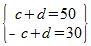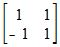# Solving Systems of Linear Equations in Two Variables Using Determinants

An error occurred trying to load this video.

Try refreshing the page, or contact customer support.

Coming up next: Solving Systems of Linear Equations in Three Variables Using Determinants

### You're on a roll. Keep up the good work!

Replay
Your next lesson will play in 10 seconds
• 0:02 Linear System in Two Variables
• 1:12 Cramer's Rule
• 2:24 Finding the Determinants
• 3:32 Finding the Solution
• 4:06 Lesson Summary

Want to watch this again later?

Timeline
Autoplay
Autoplay
Speed

#### Recommended Lessons and Courses for You

Lesson Transcript
Instructor: Yuanxin (Amy) Yang Alcocer

Amy has a master's degree in secondary education and has taught math at a public charter high school.

Watch this video lesson to learn how you can easily find the solution of a linear system in two variables by just using determinants. All you need to find the complete solution is to find three determinants.

## Linear System in Two Variables

Linear systems in two variables are common in math. You will see them more and more as you progress. They are collections of two equations with two variables and no exponents. There are several methods that you can use to solve these systems.

We will talk about the method that uses matrices and determinants in this video lesson. Take a moment to refresh your memory, if you need, on how to create matrices from equations and also how to find the determinants of matrices.

What kind of a problem might you expect to solve? For example, linear systems in two variables can solve such a problem as how many dogs and cats there are at a pet store.

Say a pet store owner tells you he knows that he has a total of 50 dogs and cats, and he knows that when he subtracts the number of cats from dogs he gets 30. Well, you can totally help him figure out how many cats and dogs he has by creating this linear system in two variables:The first equation tells you that when you add the number of cats and dogs together you get a total of 50. The second equation tells you that when you subtract the number of cats from dogs you get 30. This is your system.

## Cramer's Rule

To help you solve this system, we can use Cramer's rule. Cramer's rule tells you how you can solve the linear system by working with just determinants. First, you create your coefficient matrix, which includes only the coefficients on the left side of the equations. Then you find the determinant of this matrix.

We will call this determinant D. Then for each variable, you take your coefficient matrix and substitute the constant numbers, which are the numbers on the right side of the equation, into each column.

So, for the c variable, you substitute the constant numbers into the first column of the coefficient matrix. For the d variable, you substitute the constant numbers into the second column of the coefficient matrix.

You then find the determinants of these matrices. For the c variable, we will call the determinant D sub c. For the d variable, we will call the determinant D sub d.

The solution then is found by division. The c solution is c = D sub c / D. The d solution is d = D sub d / D. Because we have two variables, we need to find three determinants: one general determinant and then one determinant for each variable.

## Finding the Determinants

Let's look at it in action now. We first create our coefficient matrix:To unlock this lesson you must be a Study.com Member.

### Register to view this lesson

Are you a student or a teacher?

### Unlock Your Education

#### See for yourself why 30 million people use Study.com

##### Become a Study.com member and start learning now.
Back
What teachers are saying about Study.com

### Earning College Credit

Did you know… We have over 160 college courses that prepare you to earn credit by exam that is accepted by over 1,500 colleges and universities. You can test out of the first two years of college and save thousands off your degree. Anyone can earn credit-by-exam regardless of age or education level.